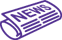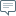### Space CareersSpace News

### Keyword

• Home
• News
• A practical method to improve the accuracy of orbit prediction and position error covariance prediction

## A practical method to improve the accuracy of orbit prediction and position error covariance prediction

Written by  Monday, 03 April 2023 20:02Write a comment

With continuous developments in the space industry, the space near the Earth is occupied by a variety of spacecraft whose number is increasing dramatically every year. To avoid a collision, huge computation power is necessary to determine the possibility of a collision between two space objects. However, there are various uncertainties in the collision prediction process, which aggravates the burdens on space safety management.

Since the collision probability is usually applied to evaluate a dangerously close encounter, improving the precision of orbit prediction and covariance prediction is key.

In a research paper recently published in Space: Science & Technology, Zhaokui Wang, from Tsinghua University, proposed an efficient method with a back propagation (BP) neural network to improve the accuracy of orbit prediction and position error covariance prediction of space targets.

Wang's team also applied the proposed method to estimate the collision probability for the Q-Sat and space debris with NORAD ID of 49863.

Q-Sat denoted the Tsinghua Gravitational and Atmospheric Science Satellite, which was a spherical microsatellite developed by Distributed and Intelligent Space System Lab (DSSL) and dedicating to the Earth's gravity field recovery and atmosphere density detection.

Firstly, the author introduced the collision analysis model. In the collision analysis, orbit prediction and covariance prediction were conducted according to the initial states and the initial covariances of two space objects. By utilizing an appropriate algorithm, the time instance where the distance between the two objects was the smallest could be obtained.

Thus, the collision probability could be derived by combining the predicted state vectors and the predicted covariances of the two objects at that time. Next, the author developed the optimized atmospheric model. The first step was to choose the atmospheric density model and its correction parameters.

In an empirical atmospheric density model, parameters such as solar activity and geomagnetic activity were employed to describe the atmospheric status. For selection of atmospheric density model to be optimized, it was necessary to consider the sensitivity of the parameters in the model, and the performance of the model in terms of orbit prediction. At present, commonly used empirical atmospheric density models were the family of Jacchia models and MSISE models.

For orbits below 500 km, the JB2008 and Jacchia models performed better in tasks of atmosphere density prediction and orbit prediction. Therefore, the Jacchia-Roberts model was selected as the atmospheric density model to be optimized. The atmospheric temperature as well as the sensitivity matrix in terms of atmospheric drag was mainly considered in the optimization process.

The second step was to applied the dynamic inversion method to optimize parameters in the selected empirical atmosphere density models. In order to test the performances of the atmospheric density model correction method, a total of five-day orbit data of Q-sat from January 11 to January 15, 2022 were selected.

Among them, every 24-hour orbit is employed as a correction unit. To examine the accuracy of the optimized Jacchia-Roberts model, the NRLMSISE-00 model is employed for comparison. The 24-hour prediction position error by using the modified Jacchia-Roberts model is about 65m lower than the prediction by using the NRLMSISE-00 model. Compared to the original Jacchia-Roberts model, the averaged 24-hour prediction accuracy over 14 days is elevated by approximately 170m.

Afterwards, the author employed back propagation (BP) neural network to predict the position error covariance of the Q-Sat and the space debris. The learning rule of the BP neural network was to use the gradient descent method to adjust the weights and thresholds of the network through reverse propagation.

The same method was used to minimize the sum of squares of network errors. It was proved that the three-layer neural network could approximate a nonlinear continuous function with arbitrary accuracy, and the approximation accuracy was higher than a polynomial method.

However, the accuracy of the BP neural network highly depended on the quality of the sample data. In this investigation, a large number of covariance datasets were employed for the BP neural network training. Fifty groups of high-precision orbit data of the Q-Sat from November 2021 to January 2022 were used.

An orbit prediction model was employed to predict the orbit of the satellite. The deviation between the predicted ephemeris and the precise ephemeris were obtained. It could be observed that the Q-Sat position prediction errors were increased with time. The longer the prediction time, the higher the prediction errors. Errors in T-directions were the largest among the errors in the three directions. The position prediction errors were employed to train the BP neural network.

After backpropagating the errors from the output layer toward the input layer, an accurate nonlinear relationship between input and output could be established. When it came to BP neural network for predicting position errors for the space debris, usually two-line elements (TLE) data was the only representation of its orbital behavior.

The TLE data, however, did not include the errors for the entire orbital period. Thus, the orbit from half orbital period before and after a time epoch was considered as a reference. The SGP4 was used to predict the orbit based on TLE data.

The TLE data of the space debris with NORAD ID of 49863 249 from November 2021 to January 2022 were selected for obtaining the BP neural network training 250 data. According to the actual prediction errors, designed BP was reliable for predicting a space debris position prediction covariance with TLE data.

Finally, the author presented and discussed their own simulation results and the reported results for the dangerous encounter between the Q-Sat and Space Debris. It was reported that the Q-Sat would collide with space debris on January 18, 2022, the collision probability was about 3.71×10−4. According to the author's investigation, the nearest distance between Q-Sat and Space Debris was 2.71 282 km and the collision probability was 1.16 × 10–11. It was determined that the warning of the encounter was actually a false alarm.

The results also showed that the proposed method could improve the fidelity of object collision prediction. The improvement of collision warning fidelity relied on long-term high-quality tracking data. By equipping precise determination devices on satellites, the prediction accuracy of with would be greatly improved, and the number of unnecessary avoidance maneuvers would be reduced.

More information: Huang Pu et al, Reduction of Space Debris Collision Prediction Uncertainty Based on Q-Sat Precise Orbit, Space: Science & Technology (2023). DOI: 10.34133/space.0005

Provided by Beijing Institute of Technology Press Co., Ltd

Citation: A practical method to improve the accuracy of orbit prediction and position error covariance prediction (2023, April 3) retrieved 4 April 2023 from https://phys.org/news/2023-04-method-accuracy-orbit-position-error.html
This document is subject to copyright. Apart from any fair dealing for the purpose of private study or research, no part may be reproduced without the written permission. The content is provided for information purposes only.Be the first to comment.

### CONTACT THE AUTHOR

* Denotes Required Field
Personal information
Message

### Interested in Space?

Hit the buttons below to follow us...